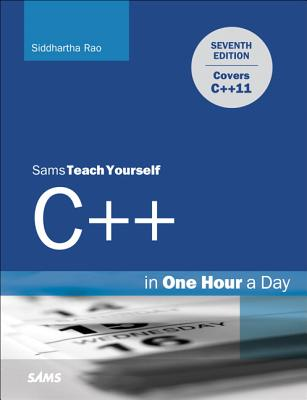### Sams Teach Yourself C++ in One Hour a Day### Huge savings for students

Each student receives a 50% discount off of most books in the HSG Book Store. During class, please ask the instructor about purchase details.
 List Price: \$44.99 Price: \$22.50 You Save: \$22.50
1Learn C++ in Just One Hour a Day

Completely updated for the C++11 standard, Sams Teach Yourself C++ in One Hour a Day presents the language from a practical point of view, helping you learn how to use C++11 to create faster, simpler, and more efficient C++ applications.

• Master the fundamentals of C++ and object-oriented programming
• Understand how C++11 features help you write compact and efficient code using concepts such as lambda expressions, move constructors, and assignment operators
• Learn the Standard Template Library, including containers and algorithms used in most real-world C++ applications
• Test your knowledge and expertise using exercises at the end of every lesson

• No previous programming experience required
• Learn C++11, object-oriented programming, and analysis
• Write fast and powerful C++ programs, compile the source code with a gcc compiler, and create executable files
• Use the Standard Template Library's (STL) algorithms and containers to write feature-rich yet stable C++ applications
• Develop sophisticated programming techniques using lambda expressions, smart pointers, and move constructors
• Learn to expand your program's power with inheritance and polymorphism
• Master the features of C++ by learning from programming experts
• Learn C++11 features that allow you to program compact and high-performance C++ applications

PART I: THE BASICS

LESSON 1: Getting Started with C++11

LESSON 2: The Anatomy of a C++ Program

LESSON 3: Using Variables, Declaring Constants

LESSON 4: Managing Arrays and Strings

LESSON 5: Working with Expressions, Statements, and Operators

LESSON 6: Controlling Program Flow

LESSON 7: Organizing Code with Functions

LESSON 8: Pointers and References Explained

PART II: FUNDAMENTALS OF OBJECT-ORIENTED C++ PROGRAMMING

LESSON 9: Classes and Objects

LESSON 10: Implementing Inheritance

LESSON 11: Polymorphism

LESSON 13: Casting Operators

LESSON 14: An Introduction to Macros and Templates

PART III: LEARNING THE STANDARD TEMPLATE LIBRARY (STL)

LESSON 15: An Introduction to the Standard Template Library

LESSON 16: The STL String Class
LESSON 17: STL Dynamic Array Classes
LESSON 18: STL list and forward_list
LESSON 19: STL Set Classes
LESSON 20: STL Map Classes

PART IV: MORE STL

LESSON 21: Understanding Function Objects

LESSON 22: C++11 Lambda Expressions
LESSON 23: STL Algorithms
LESSON 24: Adaptive Containers: Stack and Queue
LESSON 25: Working with Bit Flags Using STL

LESSON 26: Understanding Smart Pointers

LESSON 27: Using Streams for Input and Output
LESSON 28: Exception Handling
LESSON 29: Going Forward

APPENDIXES

A: Working with Numbers: Binary and Hexadecimal

B: C++ Keywords

C: Operator Precedence